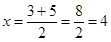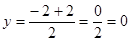NCERT Solutions Maths Class 10 Exercise 7.3

# NCERT Solutions Maths Class 10 Exercise 7.3

## Maths Class 10 Exercise 7.3

1. Find the area of the triangle whose vertices are:

(i) (2, 3), (–1, 0), (2, –4)

(ii) (–5, –1), (3, –5), (5, 2)

Solution: (i) The vertices of the triangle are (2, 3), (–1, 0), (2, –4).

= ½ [2{0 − (−4)} – 1(−4 − 3) + 2(3 − 0)]

= ½ [2(0 + 4) – 1(−7) + 2(3)]

½ (8 + 7 + 6) = 21/2 sq. units

(ii) The vertices of the triangle are (–5, –1), (3, –5), (5, 2).

= ½ [−5(−5 − 2) + 3{2 − (−1)} + 5{−1 − (−5)}]

= ½ [−5(−7) + 3(3) + 5(4)]

= ½ (35 + 9 + 20)

= ½ (64)

= 32 sq. units

2. In each of the following, find the value of ‘k’, for which the points are collinear.

(i) (7, –2), (5, 1), (3, k)

(ii) (8, 1), (k, –4), (2, –5)

Solution: (i) The vertices of the triangle are (7, –2), (5, 1), (3, k).

Since the given points are collinear, no triangle will be formed and hence the area of the triangle formed by them is equal to zero.

½ [7(1 − k) + 5{k − (−2)} + 3(−2 − 1)] = 0

½ (7 − 7k + 5k + 10 − 9) = 0

½ (7 − 7k + 5k + 1) = 0

½ (8 − 2k) = 0

8 − 2k = 0

2k = 8

k = 4

(ii) The vertices of the triangle are (8, 1), (k, –4), (2, –5).

Since the given points are collinear, no triangle will be formed and hence the area of the triangle formed by them is equal to zero.

½ [8{−4 − (−5)} + k(−5 − 1) + 2{1 − (−4)}] = 0

= ½ (8 − 6k + 10) = 0

½ (18 − 6k) = 0

18 − 6k = 0

18 = 6k

k = 3

3. Find the area of the triangle formed by joining the mid-points of the sides of the triangle whose vertices are (0, –1), (2, 1) and (0, 3). Find the ratio of this area to the area of the given triangle.

Solution: Let A(0, –1) = (x1, y1), B(2, 1) = (x2, y2) and C(0, 3) = (x3, y3) are the vertices of the given triangle.

Area of ABC = ½ [0(1 − 3) + 2{3 − (−1)} + 0(−1 − 1)] = ½ × 8

= 4 sq. units

Let P, Q and R be the mid-points of the sides AB, AC and BC respectively.

Applying the section formula to find the coordinates of P, Q and R, we get

Applying the same formula to find the area of PQR.

Area of PQR = ½ [1(1 − 2) + 0(2 − 0) + 1(0 − 1)] = ½ (−2)

= 1 sq. units (Neglecting the minus sign)

4. Find the area of the quadrilateral whose vertices, taken in order, are (–4, –2), (–3, –5), (3, –2) and (2, 3).

Solution: Area of quadrilateral ABCD = Area of triangle ABD + Area of triangle BCD ….. (i)

Using the formula to find the area of triangle ABD:

= ½ [−4(−5 − 3) – 3{3 − (−2)} + 2{−2 − (−5)}]

= ½ (32 – 15 + 6)

= ½ (23) = 11.5 sq. units      …… (ii)

Again, using the formula to find the area of triangle BCD:

= ½ [−3(−2 − 3) + 3{3 − (−5)} + 2{−5 − (−2)}]

= ½ (15 + 24 − 6)

= ½ (33) = 16.5 sq. units     …… (iii)

Putting the values from equations (ii) and (iii) in equation (i), we get

Area of quadrilateral ABCD = 11.5 + 16.5 = 28 sq. units

5. We know that the median of a triangle divides it into two triangles of equal areas. Verify this result for ABC whose vertices are A(4, –6), B(3, –2) and C(5, 2).

Solution: We are given a ABC whose vertices are A(4, –6), B(3, –2) and C(5, 2), and AD is one of its medians.

We need to show that ar(ABD) = ar(ACD).

Let the coordinates of point D are (x, y).

Using the section formula to find the coordinates of point D, we getTherefore, the coordinates of point D are (4, 0).

Using the formula to find the area of triangle ABD:

= ½ [4(−2 − 0) + 3{0 − (−6)} + 4{−6 − (−2)}]

= ½ (−8 + 18 −16)

= ½ (−6)

= −3 sq. units

Therefore, area of ABD = 3 sq. units             (Area cannot be negative) …… (i)

Again using the formula to find the area of triangle ACD:

½ [4(2 − 0) + 5{0 − (−6)} + 4(−6 −2)]

½ (8 + 30 − 32)

= ½ (6)

Therefore, area of ACD = 3 sq. units     …… (ii)

From equations (i) and (ii), we get ar(ABD) = ar(ACD)

Hence Proved.